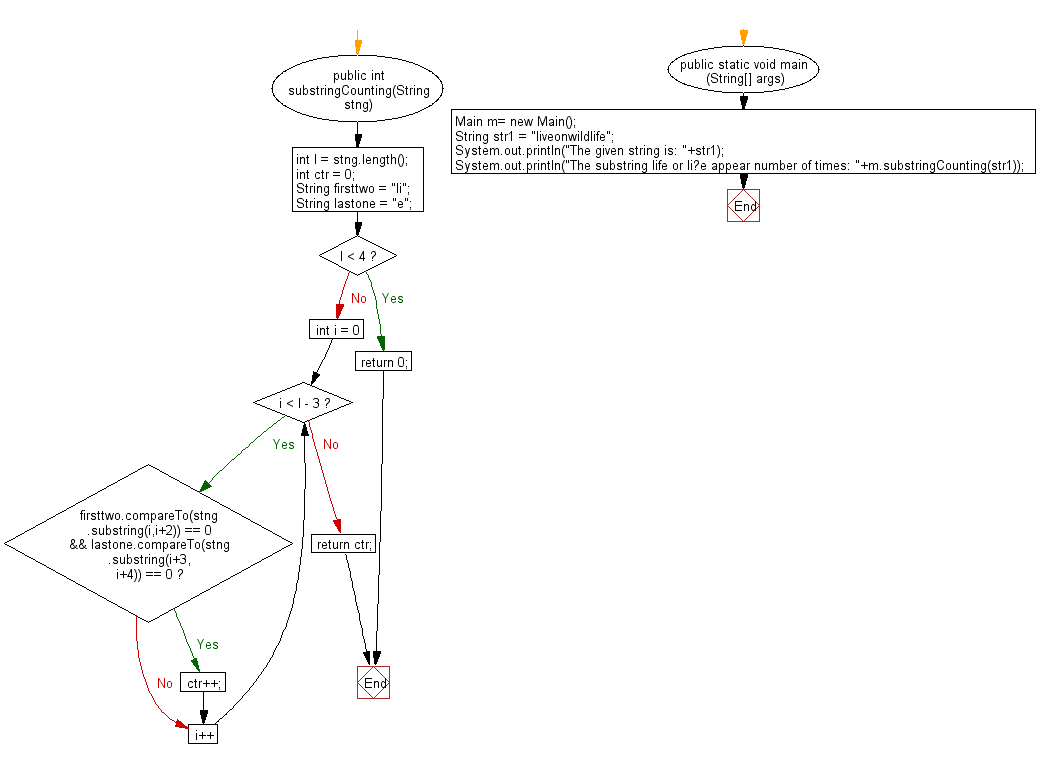﻿ Java exercises: Count how many times the substring 'life' present at anywhere in a given string - w3resource# Java String Exercises: Count how many times the substring 'life' present at anywhere in a given string

## Java String: Exercise-76 with Solution

Write a Java program to count how many times the substring 'life' present at anywhere in a given string. Counting can also happen for the substring 'li?e',any character instead of 'f'.

Sample Solution:

Java Code:

``````import java.util.*;
public class Main
{
public int substringCounting(String stng)
{
int l = stng.length();
int ctr = 0;
String firsttwo = "li";
String lastone = "e";
if (l < 4)
return 0;
for (int i = 0; i < l - 3; i++)
{
if (firsttwo.compareTo(stng.substring(i,i+2)) == 0 && lastone.compareTo(stng.substring(i+3, i+4)) == 0)
ctr++;
}
return ctr;
}
public static void main (String[] args)
{
Main m= new Main();
String str1 =  "liveonwildlife";
System.out.println("The given string is: "+str1);
System.out.println("The substring life or li?e appear number of times: "+m.substringCounting(str1));
}
}
``````

Sample Output:

```The given string is: liveonwildlife
The substring life or li?e appear number of times: 2
```

Pictorial Presentation:Flowchart:Java Code Editor:

Improve this sample solution and post your code through Disqus

What is the difficulty level of this exercise?

﻿

## Java: Tips of the Day

Count the number of occurrences of a char in a String:

```int count = StringUtils.countMatches("a.b.c.d", ".");
```

Ref: https://bit.ly/2OTy6sG